Updating search results...

# 8 Results

View
Selected filters:
• Dan MeyerUnrestricted Use
CC BY
Rating
0.0 stars

In this lesson, students explore arithmetic patterns to help solve a real-world problem involving addition operations. This lesson supports the 3rd grade standard of identifying arithmetic patterns and explaining them using properties of operations.

Subject:
Mathematics
Material Type:
Lesson Plan
Provider:
Dan Meyer
12/28/2015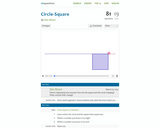Unrestricted Use
CC BY
Rating
0.0 stars

This resource could be used an a precurser for a lesson on comparing areas of circles and squares. There is guess and check involved, as well as digging deeper to find the correct answer. Students could do part of this on their own device, using GeoGebra to help with graphing the equations. If students do not have access to devices, then the teacher can use it as a class discussion.

This includes questions that can be posed to students, as well as visuals to make the entire task make sense to students. Answer is included.

Subject:
Mathematics
Material Type:
Alternate Assessment
Interactive
Simulation
Provider:
Dan Meyer
04/18/2016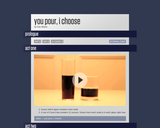Unrestricted Use
CC BY
Rating
0.0 stars

You Pour, I Choose presents students with a real-world application of which cylindrical container holds more soda. Students will find the volume of soda in two different sized cylinders.

Subject:
Mathematics
Material Type:
Diagram/Illustration
Simulation
Provider:
Dan Meyer
12/28/2015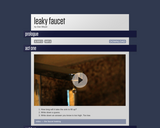Unrestricted Use
CC BY
Rating
0.0 stars

This is a 3 act lesson by Dan Meyer.  In this lesson students ask and answer, "How long does it take the sink to fill up?", after viewing a video of a leaky fauct.

Subject:
Mathematics
Material Type:
Diagram/Illustration
Lesson Plan
Simulation
Provider:
Dan Meyer
04/18/2016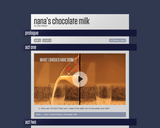Conditional Remix & Share Permitted
CC BY-NC
Rating
0.0 stars

This Three-Act task shows students a video of how Dan messed up his Nana's chocolate milk proportions. Using a double number line, students try to find ways to fix the mistake using different ratios.

Subject:
Mathematics
Material Type:
Formative Assessment
Lesson Plan
Provider:
Dan Meyer
06/16/2015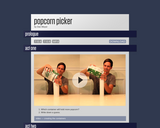Conditional Remix & Share Permitted
CC BY-NC
Rating
0.0 stars

This is a three act lesson in which students investigate the volume of 2 different cylinders made from the same size of paper. Students determine which would container would hold the greater volume of popcorn, or if the volume would be the same.

Subject:
Mathematics
Material Type:
Lesson Plan
Provider:
Dan Meyer
06/16/2015Unrestricted Use
CC BY
Rating
0.0 stars

In this Dan Meyer Three Act, students are asked to determine how many levels of pyramids can be created by a container of toothpicks.  During the next "Acts," Dan asks the students how many levels can be created by 250 toothpicks, 500 toothpicks, and concludes by having the students write a function that relates the number of levels of the pyramid to the number of toothpicks.

Subject:
Mathematics
Material Type:
Activity/Lab
Diagram/Illustration
Interactive
Lesson Plan
Simulation
Provider:
Dan Meyer
04/18/2016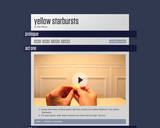Conditional Remix & Share Permitted
CC BY-NC
Rating
0.0 stars

Math in Three Acts by Dan Meyers are three part math tasks that engage students through thought provoking videos. The main question students engage with is "What's the probability of getting a yellow starburst in a package of starburst?" Students will think about what they information they need to determine this in Act 2. Student then predict the experimental probability based on the theoretical probability in Act 3.

Subject:
Mathematics
Material Type:
Diagram/Illustration